# Blog Archives

## K-fold cross-validation in Stan

July 30, 2018
By

Comparing multiple models is one of the core but also one of the trickiest element of data analysis. Under a Bayesian framework the loo package in R allows you to derive (among other things) leave-one-out cross-validation metrics to compare the predictive abilities of different models. Cross-validation is basically: (i) separating the data into chunks, (ii) Related PostAutomated Text Feature...

## Second step with non-linear regression: adding predictors

June 19, 2017
By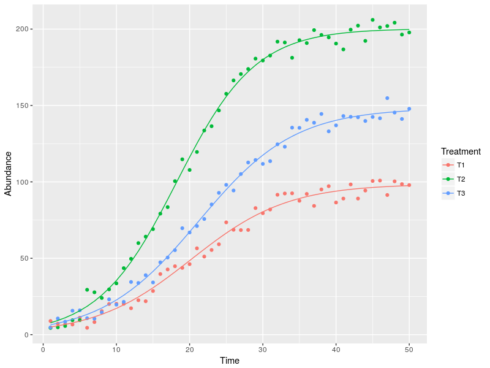In this post we will see how to include the effect of predictors in non-linear regressions. In other words, letting the parameters of non-linear regressions vary according to some explanatory variables (or predictors). Be sure to check the first post on this if you are new to non-linear regressions. The example that I will use Related Post Weather forecast with...

## Assessing significance of slopes in regression models with interaction

March 17, 2016
By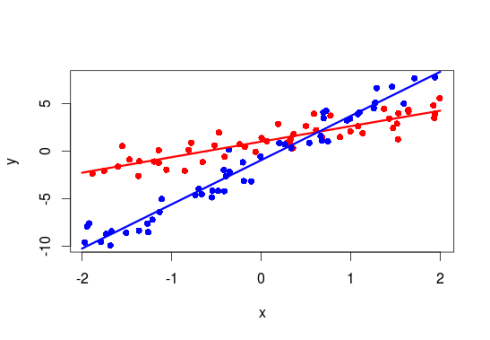This is a pretty short post on an issue that popped at some point in the past, at that time I found a way around it but as it arose again recently I decided to go through it. The issue I had was that when modeling an interaction between a continuous (say temperature) and a Related PostFirst steps with...

## Mastering R plot – Part 3: Outer margins

March 5, 2016
By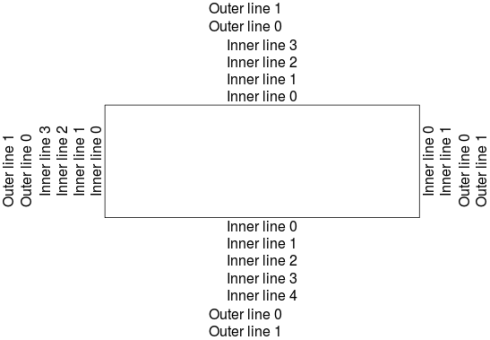This is the third post in our series Mastering R Plot, in this one we will cover the outer margins. To know more about plot customization read my first and second post. Let’s directly dive into some code: #a plot has inner and outer margins #by default there is no outer margins par()\$oma 0 Related PostInteractive plotting with...

## First steps with Non-Linear Regression in R

February 25, 2016
ByDrawing a line through a cloud of point (ie doing a linear regression) is the most basic analysis one may do. It is sometime fitting well to the data, but in some (many) situations, the relationships between variables are not linear. In this case one may follow three different ways: (i) try to linearize the

## Mastering R plot – Part 2: Axis

February 6, 2016
By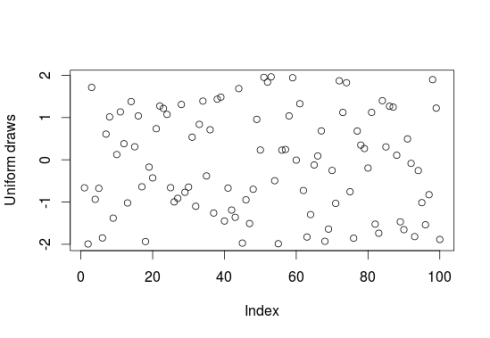This is the second part of the Mastering R plot series. The standard plot function in R allows extensive tuning of every element being plotted. There are, however, many possible ways and the standard help file are hard to grasp at the beginning. In this article we will see how to control every aspects of

## Bayesian regression with STAN Part 2: Beyond normality

January 26, 2016
By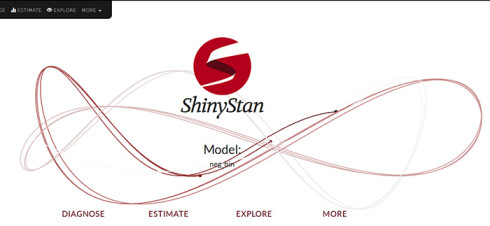In a previous post we saw how to perform bayesian regression in R using STAN for normally distributed data. In this post we will look at how to fit non-normal model in STAN using three example distributions commonly found in empirical data: negative-binomial (overdispersed poisson data), gamma (right-skewed continuous data) and beta-binomial (overdispersed binomial data).

## Mastering R Plot – Part 1: colors, legends and lines

January 19, 2016
ByThis is the first post of a series that will look at how to create graphics in R using the plot function from the base package. There are of course other packages to make cool graphs in R (like ggplot2 or lattice), but so far plot always gave me satisfaction. In this post we will

## Bayesian regression with STAN: Part 1 normal regression

January 8, 2016
By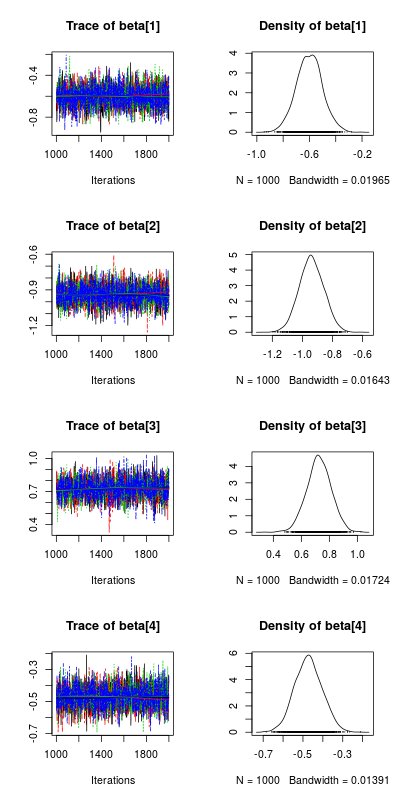This post will introduce you to bayesian regression in R, see the reference list at the end of the post for further information concerning this very broad topic. Bayesian regression Bayesian statistics turn around the Bayes theorem, which in a regression context is the following: \$\$ P(theta|Data) propto P(Data|theta) times P(theta) \$\$ Where (theta) is

## Bringing the powers of SQL into R

December 11, 2015
ByOne of the big flaw of R is that data loaded into it are stored in the memory (on the RAM) and not on the disk. As you are working in an analysis with large (big) data the processing time of simple and more complex functions can become very long or even crash your computer.